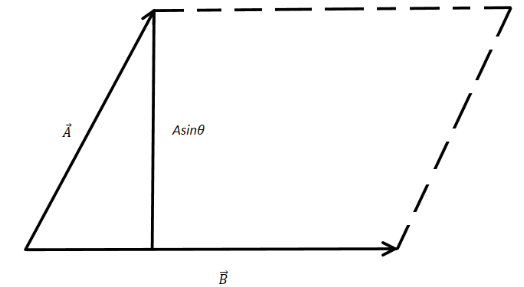Courses
Courses for Kids
Free study material
Offline Centres
MoreLast updated date: 03rd Dec 2023
Total views: 279k
Views today: 4.79k

# Calculate the area of a parallelogram formed by vectors. $\vec A = 3\hat i + 2\hat j$ $\vec B = - 3\hat i + 7\hat j$.Verified
279k+ views
Hint: Learn how to perform vector cross product of two vectors. Learn the physical significance of the vector cross product to solve this problem. The vector cross product produces a vector which is perpendicular to both the vectors.

Formula used:
The vector cross product of two Cartesian vectors is given by,
$\vec A \times \vec B = \left| {\begin{array}{*{20}{c}} {\hat i}&{\hat j}&{\hat k} \\ a&b&c \\ e&f&g \end{array}} \right|$
where, $\hat i$ ,$\hat j$and $\hat k$ are the unit vectors along X,Y,Z respectively $a,b,c$ are the components of $\vec A$ and $e,f,g$ are $t$.

We have given here two vectors, $\vec A = 3\hat i + 2\hat j$ and $\vec B = - 3\hat i + 7\hat j$. Now, the cross product of two vectors produces a new vector which is perpendicular to both the vectors and the magnitude of it is nothing but the area of a parallelogram of sides with the magnitude of the vectors. Since, the sine component of the vector resembles the height of the parallelogram made by the vectors. Hence, the magnitude of the cross product is the area of the parallelogram.Now, the vector cross product of two Cartesian vectors is given by, $\vec A \times \vec B = \left| {\begin{array}{*{20}{c}} {\hat i}&{\hat j}&{\hat k} \\ a&b&c \\ e&f&g \end{array}} \right|$
where, $\hat i$ $\hat j$ $\hat k$ are the unit vectors along X,Y,Z respectively $a,b,c$ are the components of $\vec A$and $e,f,g$are the components of $\vec B$ along the axes.Hence, for $\vec A = 3\hat i + 2\hat j$ and $\vec B = - 3\hat i + 7\hat j$ the cross product will be,
$\vec A \times \vec B = \left| {\begin{array}{*{20}{c}} {\hat i}&{\hat j}&{\hat k} \\ 3&2&0 \\ { - 3}&7&0 \end{array}} \right|$
$\Rightarrow \vec A \times \vec B = \hat i(0 - 0) - \hat j(0 - 0) + \hat k\{ 21 - ( - 6)\}$
$\Rightarrow \vec A \times \vec B = 27\hat k$
$\therefore \left| {\vec A \times \vec B} \right| = 27$

Hence, the area of the parallelogram will be $27sq.\,unit$.

Note: The area of the parallelogram signifies a surface in the plane of the vectors which is actually a surface vector. The direction of any surface vector is always perpendicular to the surface; the direction is $\vec A \times \vec B$ here is also perpendicular to the surface of the parallelogram. When calculating the value of the determinant keep in mind that the formula is for any vector in 3D but the vectors given here are 2D vectors hence, make sure to make the Z components of the vectors to be zero.